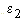# Source Code Description: Subroutine DSHELL

DSHELL

PURPOSE: to calculate the mutual impedance term contributed by the dielectric insulation on the surface of a thin wire.

METHOD: The contribution to the impedance matrix is calculated utilizing the equation belowwhere zmn is defined in subroutine SGANT,is the dielectric constant of the insulation, b is the outer radius of the insulation, a is the inner radius,is the dielectric constant of the external medium, and F is the sinusoidal expansion function.

CALLED BY: SGANT

CALLS TO: NONE